# 基于Bragg光栅的电力电缆温度在线测量方法研究Research on Online Measurement Method of Power Cable Temperature Based on Bragg Grating

DOI: 10.12677/JSTA.2020.82007, PDF, HTML, XML, 下载: 222  浏览: 596  科研立项经费支持

Abstract: Based on the principle of temperature measurement of fiber Bragg grating, the online monitoring method for power cable temperature is studied. Through ANSYS finite element simulation, the inner temperature cloud diagram of the cable is obtained and the temperature rise equation of cable core temperature and skin temperature is established. Compared with IEC-60287 standard, it is proved that the temperature rise equation is more accurate, according to the experimental schematic diagram, to select the appropriate device and build the experimental system.

1. 引言

2. 光纤Bragg光栅的测温原理

${\lambda }_{B}=2{n}_{eff}\cdot \Lambda$ (1)Figure 1. Sensing principle of fiber Bragg grating

$\Delta {\lambda }_{B}=2{n}_{eff}\Lambda \left(\frac{\Delta {n}_{eff}}{\Delta T{n}_{eff}}+\frac{\Delta \Lambda }{\Delta T\Lambda }\right)\Delta T$ (2)

$K={\lambda }_{B}\left(\frac{\Delta {n}_{eff}}{\Delta T{n}_{eff}}+\frac{\Delta \Lambda }{\Delta T\Lambda }\right)\Delta T=\frac{\Delta {\lambda }_{B}}{\Delta T}$ (3)

$\Delta {\lambda }_{B}=K\cdot \Delta T$ (4)

3. 电力电缆热路模型法

IEC-60287标准  提供了具有代表性的电缆缆芯温度计算方法。缆芯发出的热量在扩散过程中，要经过除导体以外的所有结构；介质损耗产生的热量则要经过金属屏蔽层、铠装层、外护套等几部分；金属屏蔽损耗产生的热量要经过铠装层和外护套，而铠装损耗所产生的热量则只经过外护套。根据电力电缆的传热分析，得出其热路模型如图2所示 。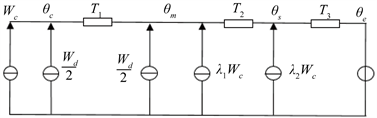Figure 2. Power cable hot circuit model

$\begin{array}{c}{\theta }_{c}={\theta }_{e}+\left[\left(1+{\lambda }_{1}+{\lambda }_{2}\right)\ast {T}_{3}+\left(1+{\lambda }_{1}\right)\ast {T}_{2}+{T}_{1}\right]\ast {W}_{c}\\ \text{\hspace{0.17em}}\text{\hspace{0.17em}}+\left[\left(1+{\lambda }_{1}+{\lambda }_{2}\right)\ast {T}_{3}+{T}_{2}+0.5\ast {T}_{1}\right]{W}_{d}\end{array}$ (5)

4. 电力电缆的仿真分析法Table 1. Cable material parameters

(1) 电缆横截面为标准圆形；

(2) 电缆材料参数分布均匀且属性不变；

(3) 边界温度均匀分布，设定为定值。

(4) 电缆层间紧密连接，忽略接触电阻。Table 2. Cable structure parameters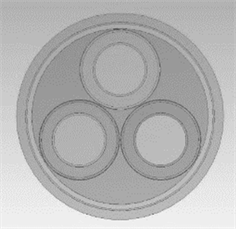Figure 3. Geometric model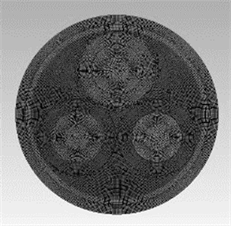Figure 4. Finite element of three-core cablemeshing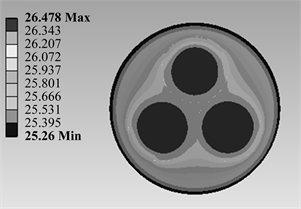Figure 5. Temperature cloud image of three-core cableTable 3. Cable skin temperature and cable core temperature under different excitation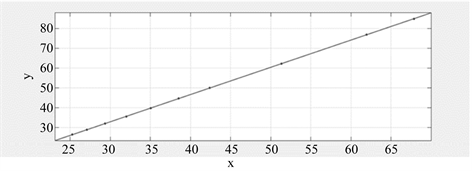Figure 6. The line diagram of skin temperature and cable core temperature was fitted

${\theta }_{c1}=1.374{\theta }_{e}-8.217$ (6)

5. 热路计算法与仿真计算法的比较

${\theta }_{c}={\theta }_{e}+\left(11.86{I}^{2}+5.73\right)×{10}^{-5}$ (7)Table 4. The results of the two formulas are compared

${T}_{1}=P\frac{{\rho }_{{T}_{1}}}{2\pi }G$ (8)

${T}_{2}=P\frac{{\rho }_{{T}_{2}}}{2\pi }{G}_{0}$ (9)

${T}_{3}=\frac{{\rho }_{{T}_{3}}}{2\pi }\mathrm{ln}\left(1+\frac{2d}{D}\right)$ (10)

$T=\frac{1}{\lambda S}$ (11)

λ为导热系数，表1中已给出；S为形状因子。

$S=\frac{2\pi }{\mathrm{ln}\left(R/r\right)}$ (12)

$S=\frac{2\pi }{\mathrm{ln}\left({r}_{2}/{r}_{1}\right)-\frac{1}{3}\mathrm{ln}\left(3{r}_{0}/{r}_{1}\right)}$ (13)Table 5. Thermal resistance coefficient modified after core temperature comparison

6. 在线温度监测系统的设计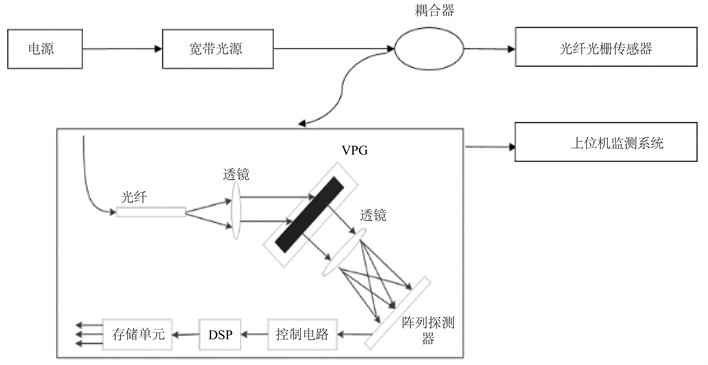Figure 7. System overall design block diagram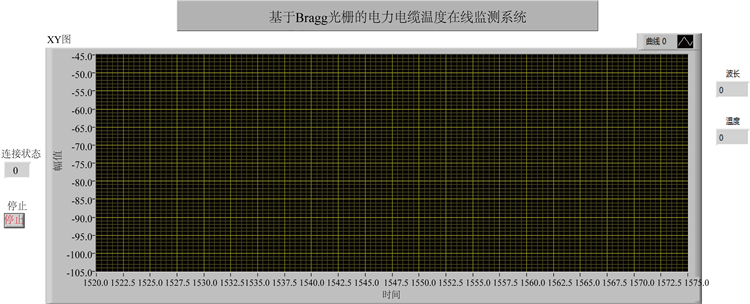Figure 8. Cable temperature monitoring interface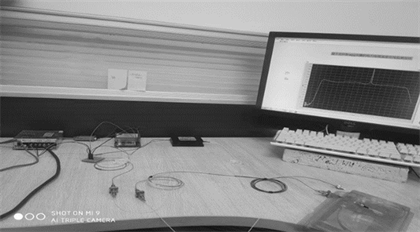Figure 9. Fiber Bragg grating temperature measurement system

7. 结束语

  杜莉. 电力电缆无线温度监测系统设计[J]. 北京工业职业技术学院学报, 2020, 19(1): 23-27.  曾含含, 程静, 张大伟, 等. 基于光纤光栅温度传感器的电缆接头温度监测系统的设计[J]. 电子测量技术, 2018, 41(6): 124-128.  贾书丽, 姚国珍. 光纤光栅传感器在电力电缆测温系统中的应用[J]. 广东电力, 2011, 24(6): 61-64.  许远标, 魏鹏. 光纤光栅温湿度检测系统研究[J]. 仪表技术与传感器, 2019(11): 70-73+77.  虢红霞. 基于光纤光栅的电力电缆温度在线监测系统研究[D]: [硕士学位论文]. 武汉: 武汉理工大学, 2010.  IEC-60287-2-1 (1994) Electric Cable-Calculation of the Current Rating-Part II: Current Rating Equations (100% Load Factor) and Calculations of Thermal Resistance. IEC Publication, 287.  赵洋洋. 基于光栅光纤传感的电力电缆在线监测系统研究[D]: [硕士学位论文]. 武汉: 武汉理工大学, 2011.  刘书铭, 王振东, 杜志叶, 等. 基于ANSYS的交联聚乙烯绝缘电缆温度场有限元计算方法[P]. 河南: CN104899379A, 2015-09-09.  李滨, 钟世敏. 结合形状因子法和平均温度差法对热力管道热损失计算的初探[J]. 机电信息, 2012(20): 41-43.  杜宁宁. 光纤Bragg光栅温度解调系统设计[D]: [硕士学位论文]. 大连: 大连理工大学, 2010.  赵勇图, 彭东林, 郑永, 王伟, 王露露. 基于小波变换与LabVIEW的时栅信号采集分析系统设计[J]. 仪表技术与传感器, 2019(8): 106-111.